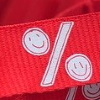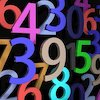# Resources tagged with: Interactivities

Filter by: Content type:
Age range:
Challenge level:

### There are 92 results

Broad Topics > Physical and Digital Manipulatives > Interactivities### Tilted Squares

##### Age 11 to 14Challenge Level

It's easy to work out the areas of most squares that we meet, but what if they were tilted?### Poly Plug Rectangles

##### Age 5 to 14Challenge Level

The computer has made a rectangle and will tell you the number of spots it uses in total. Can you find out where the rectangle is?### A Tilted Square

##### Age 14 to 16Challenge Level

The opposite vertices of a square have coordinates (a,b) and (c,d). What are the coordinates of the other vertices?### Squaring the Circle and Circling the Square

##### Age 14 to 16Challenge Level

If you continue the pattern, can you predict what each of the following areas will be? Try to explain your prediction.### Disappearing Square

##### Age 11 to 14Challenge Level

Do you know how to find the area of a triangle? You can count the squares. What happens if we turn the triangle on end? Press the button and see. Try counting the number of units in the triangle now. . . .### Square Coordinates

##### Age 11 to 14Challenge Level

A tilted square is a square with no horizontal sides. Can you devise a general instruction for the construction of a square when you are given just one of its sides?### Matter of Scale

##### Age 14 to 16Challenge Level

Prove Pythagoras' Theorem using enlargements and scale factors.### Tilting Triangles

##### Age 14 to 16Challenge Level

A right-angled isosceles triangle is rotated about the centre point of a square. What can you say about the area of the part of the square covered by the triangle as it rotates?### Isosceles Triangles

##### Age 11 to 14Challenge Level

Draw some isosceles triangles with an area of $9$cm$^2$ and a vertex at (20,20). If all the vertices must have whole number coordinates, how many is it possible to draw?### Rolling Around

##### Age 11 to 14Challenge Level

A circle rolls around the outside edge of a square so that its circumference always touches the edge of the square. Can you describe the locus of the centre of the circle?### Tessellation Interactivity

##### Age 7 to 16Challenge Level

An environment that enables you to investigate tessellations of regular polygons### Square It

##### Age 11 to 16Challenge Level

Players take it in turns to choose a dot on the grid. The winner is the first to have four dots that can be joined to form a square.### Icosian Game

##### Age 11 to 14Challenge Level

This problem is about investigating whether it is possible to start at one vertex of a platonic solid and visit every other vertex once only returning to the vertex you started at.### Sliding Puzzle

##### Age 11 to 16Challenge Level

The aim of the game is to slide the green square from the top right hand corner to the bottom left hand corner in the least number of moves.### Overlap

##### Age 11 to 14Challenge Level

A red square and a blue square overlap so that the corner of the red square rests on the centre of the blue square. Show that, whatever the orientation of the red square, it covers a quarter of the. . . .### Excel Interactive Resource: Fraction Multiplication

##### Age 11 to 16Challenge Level

Use Excel to explore multiplication of fractions.### Jam

##### Age 14 to 16Challenge Level

To avoid losing think of another very well known game where the patterns of play are similar.### Got It

##### Age 7 to 14Challenge Level

A game for two people, or play online. Given a target number, say 23, and a range of numbers to choose from, say 1-4, players take it in turns to add to the running total to hit their target.### Factor Lines

##### Age 7 to 14Challenge Level

Arrange the four number cards on the grid, according to the rules, to make a diagonal, vertical or horizontal line.### Colour in the Square

##### Age 7 to 16Challenge Level

Can you put the 25 coloured tiles into the 5 x 5 square so that no column, no row and no diagonal line have tiles of the same colour in them?### Excel Interactive Resource: Interactive Division

##### Age 11 to 16Challenge Level

Use an Excel to investigate division. Explore the relationships between the process elements using an interactive spreadsheet.### Bow Tie

##### Age 11 to 14Challenge Level

Show how this pentagonal tile can be used to tile the plane and describe the transformations which map this pentagon to its images in the tiling.### Guesswork

##### Age 14 to 16Challenge Level

Ask a friend to choose a number between 1 and 63. By identifying which of the six cards contains the number they are thinking of it is easy to tell them what the number is.### Rollin' Rollin' Rollin'

##### Age 11 to 14Challenge Level

Two circles of equal radius touch at P. One circle is fixed whilst the other moves, rolling without slipping, all the way round. How many times does the moving coin revolve before returning to P?### Fractions and Percentages Card Game

##### Age 11 to 16Challenge Level

Can you find the pairs that represent the same amount of money?### Have You Got It?

##### Age 11 to 14Challenge Level

Can you explain the strategy for winning this game with any target?### Estimating Angles

##### Age 7 to 14Challenge Level

How good are you at estimating angles?### Countdown Fractions

##### Age 11 to 16Challenge Level

Here is a chance to play a fractions version of the classic Countdown Game.### Countdown

##### Age 7 to 14Challenge Level

Here is a chance to play a version of the classic Countdown Game.### Drips

##### Age 7 to 14Challenge Level

An animation that helps you understand the game of Nim.### Interactive Number Patterns

##### Age 14 to 16Challenge Level

How good are you at finding the formula for a number pattern ?### Stars

##### Age 11 to 14Challenge Level

Can you work out what step size to take to ensure you visit all the dots on the circle?### Got a Strategy for Last Biscuit?

##### Age 11 to 16Challenge Level

Can you beat the computer in the challenging strategy game?### Nine Colours

##### Age 11 to 16Challenge Level

Can you use small coloured cubes to make a 3 by 3 by 3 cube so that each face of the bigger cube contains one of each colour?### Spot the Card

##### Age 14 to 16Challenge Level

It is possible to identify a particular card out of a pack of 15 with the use of some mathematical reasoning. What is this reasoning and can it be applied to other numbers of cards?### More Number Pyramids

##### Age 11 to 14Challenge Level

When number pyramids have a sequence on the bottom layer, some interesting patterns emerge...### Number Pyramids

##### Age 11 to 14Challenge Level

Try entering different sets of numbers in the number pyramids. How does the total at the top change?### Picturing Triangular Numbers

##### Age 11 to 14Challenge Level

Triangular numbers can be represented by a triangular array of squares. What do you notice about the sum of identical triangle numbers?### Changing Places

##### Age 14 to 16Challenge Level

Place a red counter in the top left corner of a 4x4 array, which is covered by 14 other smaller counters, leaving a gap in the bottom right hand corner (HOME). What is the smallest number of moves. . . .### Partitioning Revisited

##### Age 11 to 14Challenge Level

We can show that (x + 1)² = x² + 2x + 1 by considering the area of an (x + 1) by (x + 1) square. Show in a similar way that (x + 2)² = x² + 4x + 4### Konigsberg Plus

##### Age 11 to 14Challenge Level

Euler discussed whether or not it was possible to stroll around Koenigsberg crossing each of its seven bridges exactly once. Experiment with different numbers of islands and bridges.### Inside Out

##### Age 14 to 16Challenge Level

There are 27 small cubes in a 3 x 3 x 3 cube, 54 faces being visible at any one time. Is it possible to reorganise these cubes so that by dipping the large cube into a pot of paint three times you. . . .### Power Crazy

##### Age 11 to 14Challenge Level

What can you say about the values of n that make $7^n + 3^n$ a multiple of 10? Are there other pairs of integers between 1 and 10 which have similar properties?### Cogs

##### Age 11 to 14Challenge Level

A and B are two interlocking cogwheels having p teeth and q teeth respectively. One tooth on B is painted red. Find the values of p and q for which the red tooth on B contacts every gap on the. . . .### The Triangle Game

##### Age 11 to 16Challenge Level

Can you discover whether this is a fair game?### Just Rolling Round

##### Age 14 to 16Challenge Level

P is a point on the circumference of a circle radius r which rolls, without slipping, inside a circle of radius 2r. What is the locus of P?### Excel Interactive Resource: Haitch

##### Age 11 to 16Challenge Level

An Excel spreadsheet with an investigation.### Excel Interactive Resource: Fraction Addition & Fraction Subtraction

##### Age 11 to 16Challenge Level

Use Excel to practise adding and subtracting fractions.### More Magic Potting Sheds

##### Age 11 to 14Challenge Level

The number of plants in Mr McGregor's magic potting shed increases overnight. He'd like to put the same number of plants in each of his gardens, planting one garden each day. How can he do it?### Excel Interactive Resource: Multiples Chain

##### Age 11 to 16Challenge Level

Use an interactive Excel spreadsheet to investigate factors and multiples.